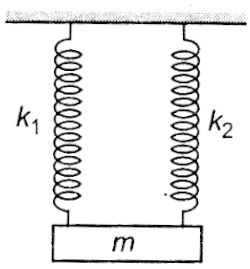A mass is suspended separately by two different springs in successive order, then time period is T1 and T2 respectively.If it is connected by both springs as shown in Fig. then time period is T0. The correct relation is

# A mass is suspended separately by two different springs in successive order, then time period is T1 and T2 respectively.If it is connected by both springs as shown in Fig. then time period is T0. The correct relation is1. A

${\mathrm{T}}_{0}^{2}={\mathrm{T}}_{1}^{2}+{\mathrm{T}}_{2}^{2}$

2. B

${\mathrm{T}}_{0}^{-2}={\mathrm{T}}_{1}^{-2}+{\mathrm{T}}_{2}^{-2}$

3. C

${\mathrm{T}}_{0}^{-1}={\mathrm{T}}_{1}^{-1}+{\mathrm{T}}_{2}^{-1}$

4. D

${\mathrm{T}}_{0}={\mathrm{T}}_{1}+{\mathrm{T}}_{2}$

Register to Get Free Mock Test and Study Material

+91

Verify OTP Code (required)

### Solution:

$\mathrm{T}=2\mathrm{\pi }\sqrt{\frac{\mathrm{m}}{\mathrm{k}}}⇒\mathrm{k}\propto \frac{1}{{\mathrm{T}}^{2}}$
In parallel combination,Register to Get Free Mock Test and Study Material

+91

Verify OTP Code (required)